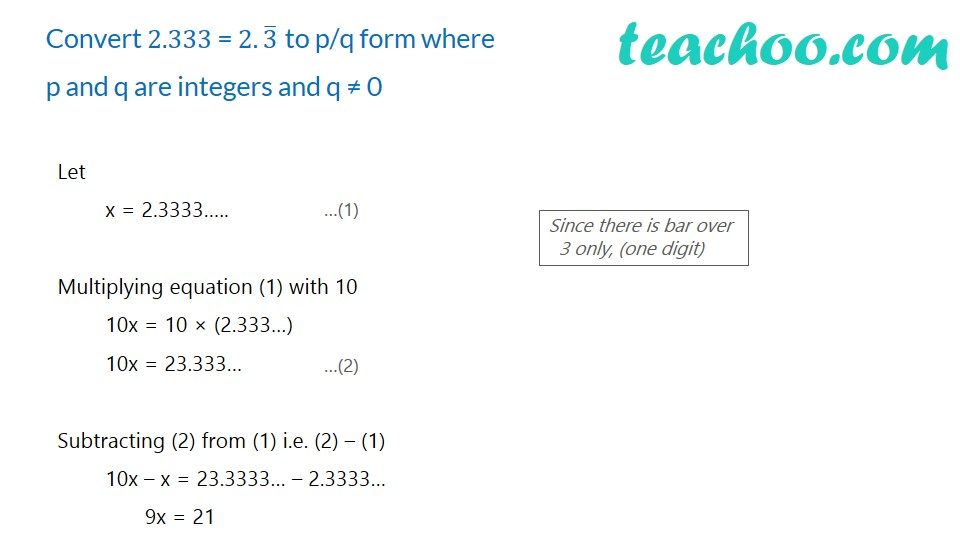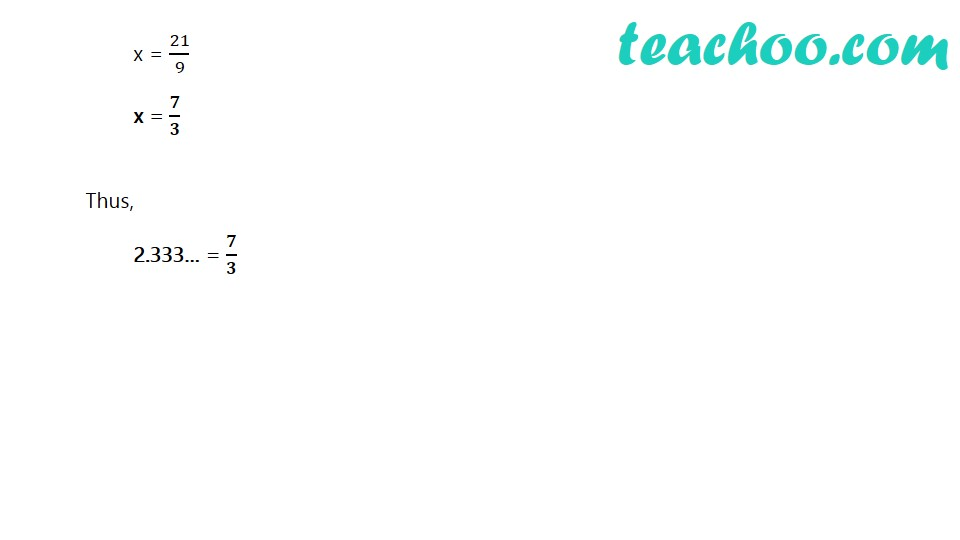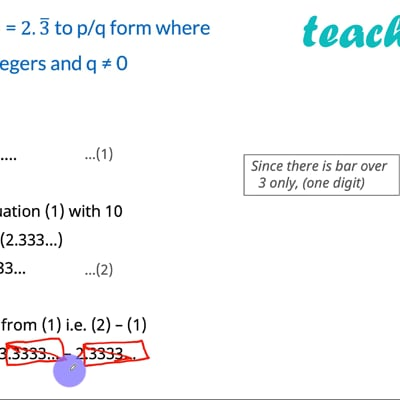Expressing decimal in p/q

Chapter 1 Class 9 Number Systems
Concept wiseThis video is only available for Teachoo black users

Solve all your doubts with Teachoo Black (new monthly pack available now!)

### Transcript

Convert 2.333 = 2.3 ̅ to p/q form where p and q are integers and q ≠ 0 Let x = 2.3333….. Multiplying equation (1) with 10 10x = 10 × (2.333…) 10x = 23.333… Subtracting (2) from (1) i.e. (2) – (1) 10x – x = 23.3333… – 2.3333… 9x = 21 Since there is bar over 3 only, (one digit) x = 21/9 x = 𝟕/𝟑 Thus, 2.333… = 𝟕/𝟑